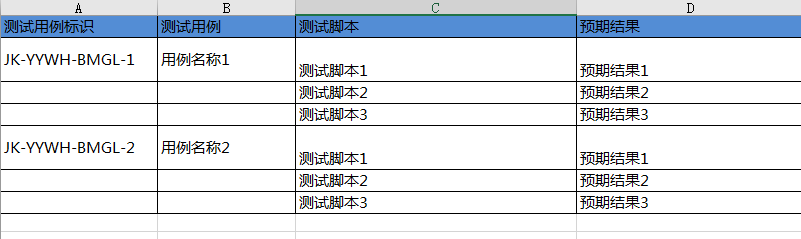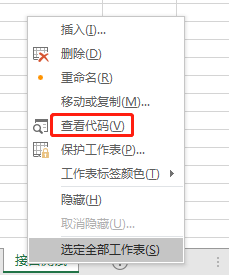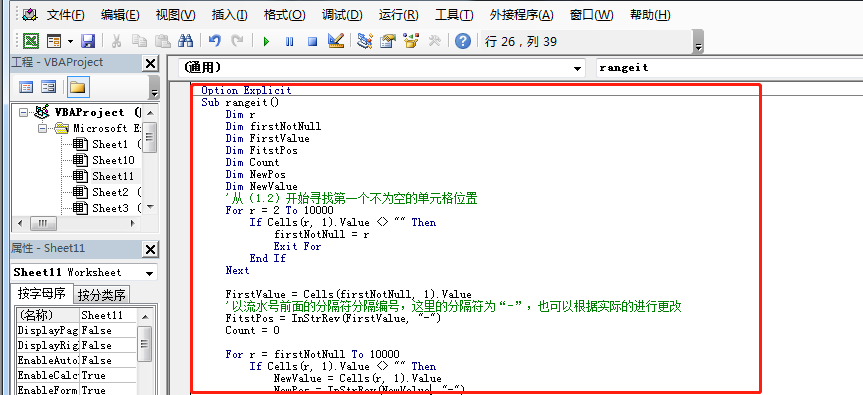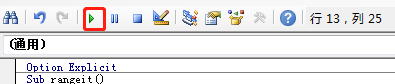• VB排序代码---7种经典排序算法已优化---可记录各种排序的时间及循环次数。
• 快速排序vb代码： Dim a() As Integer '定义一个动态数组 Sub qsort(a() As Integer, ByVal kaishi As Integer, ByVal jieshu As Integer) Dim temp As Integer, j As Integer, i As Integer i = kaishi: j = jieshu...
快速排序vb代码： Dim a() As Integer '定义一个动态数组 Sub qsort(a() As Integer, ByVal kaishi As Integer, ByVal jieshu As Integer) Dim temp As Integer, j As Integer, i As Integer i = kaishi: j = jieshu '将i、j作为指针，从两侧向中部移动 If kaishi < jieshu Then '控制是否进入循环 temp = a(kaishi) '将数组第一个值赋给temp，暂时充当对比量 While i < j Do While i < j '指针j从右向左移动，当遇到比temp小的数时，将该值移动到指针i的位置，并使i向右移动一位 If a(j) <= temp Then a(i) = a(j): i = i + 1: Exit Do j = j - 1 Loop Do While i < j '指针i从左向右移动，当遇到比temp大的数时，将该值移动到指针j的位置，并使j向左移动一位 If a(i) > temp Then a(j) = a(i): j = j - 1: Exit Do i = i + 1 Loop Wend a(i) = temp Call qsort(a(), kaishi, i - 1) '递归二分法过程进行排序 Call qsort(a(), i + 1, jieshu) Else Exit Sub End If End Sub Private Sub Command1_Click() Me.Cls Dim n As Integer, u As Integer, l As Integer n = Val(Text1) '令n=文本框中的值 ReDim a(n - 1) As Integer '重新定义数组 u = UBound(a): l = LBound(a) '得到数组的上下标 Randomize '给数组随机赋值 For i = l To u '输出排序前的数组 a(i) = Rnd * 90 + 10 Print a(i); Next Print vbCr End Sub Private Sub Command2_Click() Dim u As Integer, l As Integer u = UBound(a): l = LBound(a) Call qsort(a, l, u) '对数组使用二分法 For i = l To u '输出排序后的数组 Print a(i); Next End Sub
展开全文• VB代码 Option Explicit Sub rangeit() Dim r Dim firstNotNull Dim FirstValue Dim FitstPos Dim Count Dim NewPos Dim NewValue '从（1.2）开始寻找第一个不为空的单元格位置 For r = 2 To 10000...
工作时碰到的问题：用例模板如下，若需要在中间新增用例，就需要重新排序。因为中间有空白行，不能直接使用下拉自动填充序列功能；手动变更时，太耗费时间，还容易出错。解决方案：使用vb代码进行自动排序。
Option Explicit
Sub rangeit()
Dim r
Dim firstNotNull
Dim FirstValue
Dim FitstPos
Dim Count
Dim NewPos
Dim NewValue
'从（1.2）开始寻找第一个不为空的单元格位置
For r = 2 To 10000
If Cells(r, 1).Value <> "" Then
firstNotNull = r
Exit For
End If
Next

FirstValue = Cells(firstNotNull, 1).Value
'以流水号前面的分隔符分隔编号，这里的分隔符为“-”，也可以根据实际的进行更改
'InStrRev(),返回一个字符串在另一个字符串中首次出现的位置。搜索从字符串的末端开始，但是返回的位置是从字符串的起点开始计数的
FitstPos = InStrRev(FirstValue, "-")
Count = 0

For r = firstNotNull To 10000
If Cells(r, 1).Value <> "" Then
NewValue = Cells(r, 1).Value
NewPos = InStrRev(NewValue, "-")
If Left(FirstValue, FitstPos) <> Left(NewValue, NewPos) Then
FirstValue = NewValue
FitstPos = NewPos
Count = 0
End If
Count = Count + 1
'对编号列按流水号重新赋值
Cells(r, 1).Value = Left(FirstValue, FitstPos) & Count
End If
Next
End Sub

excel运行VB的方法：
1、选中sheet表，右键选中查看代码2、在打开的窗口中编写代码，或者在外面写好直接复制进去3、点击运行，重新排序成功展开全文• ## vb内部排序7大算法

千次阅读 2019-12-22 14:07:26
信息技术 VB程序整理排序算法（以数组a(n)降序为例）1、冒泡排序（Bubble Sort）1.0 性质总结1.1 基本思想1.2 具体步骤1.3 代码实现1.4改进的冒泡排序2、直接选择排序(Straight Select Sort)2.0 性质总结2.1 基本...


信息技术 VB程序整理
排序算法（以数组a(n)降序为例）1、冒泡排序（Bubble Sort）1.0 性质总结1.1 基本思想1.2 具体步骤1.3 代码实现1.4改进的冒泡排序
2、直接选择排序(Straight Select Sort)2.0 性质总结2.1 基本思想2.2 具体步骤2.3 代码实现
3、直接插入排序(Straight Insertion Sort)3.0 性质总结3.1 基本思想3.2 具体步骤3.3 代码实现
4、希尔排序(Shell Sort)4.0 性质总结4.1 基本思想4.2具体步骤4.3 代码实现
5、快速排序(Quick Sort)5.0 性质总结5.1 基本思想5.2 具体步骤5.3 代码实现
6、归并排序(Merge Sort)6.0 性质总结6.1 基本思想6.2 具体步骤6.3 代码实现
7、堆排序(Heap Sort)7.0 性质总结7.1 基本思想7.2 具体步骤7.3 代码实现

版权声明：本文为CSDN博主「OrganicNeptune」的原创文章，遵循 CC 4.0 BY-SA 版权协议，转载请附上原文出处链接及本声明。
原文链接：https://blog.csdn.net/OrganicNeptune/article/details/103652875

排序算法（以数组a(n)降序为例）
初始化变量值
Option Explicit
Const n = 8	'n为要排序数组元素个数
Dim a(1 To n + 1) As Integer 'a(n)为待排序数组
Dim temp(1 To n + 1) As Integer	'temp(n)在归并排序里会用到

定义交换函数（在以下所有代码块中都会用到）：
Private Sub Swap(a As Integer, b As Integer)
Dim temp As Integer
temp = a: a = b: b = temp
End Sub

1、冒泡排序（Bubble Sort）
1.0 性质总结
时间复杂度：
平均情况：O(n^2)最好情况：O(n)最坏情况：O(n^2)
空间复杂度：O(1)
稳定性：稳定
1.1 基本思想
重复的遍历待排序的数组，依次比较两个相邻的元素，若它们的顺序错误则调换位置，直至没有元素再需要交换为止。
1.2 具体步骤
比较两个相邻元素，如果前一个比后一个大，则交换这两个相邻元素从头至尾对每一对相邻元素进行步骤1的操作，完成1次对整个待排序数字列表的遍历后，最大的元素就放在了该列表的最后一个位置上了对除最后一个元素的所有元素重复上述步骤，这第二次遍历后第二大的元素就也放在了正确的位置（整个列表的倒数第二位置上）不断重复上述步骤，每次遍历都会将一个元素放在正确的位置上，从而下次遍历的元素也会随之减少一个，直至没有任何一对数字需要比较
1.3 代码实现
Private Sub BubbleSort(n As Integer)
Dim i As Integer
Dim J As Integer
For i = 1 To n - 1
For J = n To i + 1 Step -1
If a(J) > a(J - 1) Then Call Swap(a(J), a(J - 1))
Next J
Next i
End Sub

1.4改进的冒泡排序
Private Sub ImprovedBubbleSort(n As Integer)
Dim i As Integer
Dim J As Integer
Dim Flag As Boolean
For i = 1 To n - 1
Flag = True
For J = n To i + 1 Step -1
If a(J) > a(J - 1) Then Call Swap(a(J), a(J - 1)): Flag = False
Next J
If Flag = True Then Exit For
Next i
End Sub

2、直接选择排序(Straight Select Sort)
2.0 性质总结
时间复杂度：
平均情况：O(n^2)最好情况：O(n^2)最坏情况：O(n^2)
空间复杂度：O(1)
稳定性：不稳定
2.1 基本思想
先在待排序列表中找到最小（大）的元素，把它放在起始位置作为已排序序列；然后，再从剩余待排序序列中找到最小（大）的元素放在已排序序列的末尾，以此类推，直至完毕。
2.2 具体步骤
初始状态整个待排序序列为无序序列，有序序列为空每次遍历无序序列将最小元素交换到有序序列之后n-1趟遍历后排序完成
2.3 代码实现
Private Sub StraightSelectSort(n As Integer)
Dim i As Integer
Dim J As Integer
Dim k As Integer
For i = 1 To n - 1
k = i
For J = i + 1 To n
If a(k) < a(J) Then k = J
Next J
If k <> i Then Call Swap(a(k), a(i))
Next i
End Sub

3、直接插入排序(Straight Insertion Sort)
3.0 性质总结
时间复杂度：
平均情况：O(n^2)最好情况：O(n)最坏情况：O(n^2)
空间复杂度：O(1)
稳定性：稳定
3.1 基本思想
对于未排序元素，在已排序序列中从后向前扫描，找到相应位置把它插入进去；在从后向前扫描过程中，需要反复把已排序元素逐步向后挪位，为新元素提供插入空间。
3.2 具体步骤
从第一个元素开始，默认该元素已被排好序取出下一个元素，在已经排序的元素序列中从后向前扫描如果该元素（已排序）大于新元素，将该元素移到下一位置重复步骤3，直到找到已排序的元素小于或者等于新元素的位置将新元素插入到该位置后重复步骤2~5
3.3 代码实现
Private Sub StraightInsertionSort(Lside As Integer, Rside As Integer)
Dim i As Integer
Dim j As Integer
Dim tmp As Integer
For i = Lside + 1 To Rside
If a(i) > a(i - 1) Then
tmp = a(i)
For j = i - 1 To 1 Step -1
If tmp < a(j) Then Exit For
a(j + 1) = a(j)
Next j
a(j + 1) = tmp
End If
Next i
End Sub

4、希尔排序(Shell Sort)
4.0 性质总结
时间复杂度：
平均情况：O(nlogn~n^2)最好情况：O(n^1.3)最坏情况：O(n^2)
空间复杂度：O(1)
稳定性：不稳定
4.1 基本思想
将待排序列表按下标的一定增量分组（比如增量为2时，下标1，3，5，7为一组，下标2，4，6，8为另一组），各组内进行直接插入排序；随着增量的越来越小，每组所包含的数字序列越来越多，当增量减至1时，整个序列被分成一个组，排序就完成了了。
4.2具体步骤
选择一个增量序列（定义增量的递减状况，直至最后为1）按增量序列的个数k，对序列进行k趟排序每趟排序，对各分组进行直接插入排序
4.3 代码实现
Private Sub ShellSort(n As Integer)
Dim h As Integer
Dim i As Integer
Dim j As Integer
Dim temp As Integer
h = n \ 2
Do While h >= 1
For i = h + 1 To n
j = i - h
Do While j > 0
If a(j) < a(j + h) Then
Call Swap(a(j), a(j + 1))
j = j - h
Else
j = 0
End If
Loop
Next i
h = h \ 2
Loop
End Sub

5、快速排序(Quick Sort)
5.0 性质总结
时间复杂度：
平均情况：O(nlogn)最好情况：O(nlong)最坏情况：O(n^2)
空间复杂度：
平均情况：O(logn)最好情况：O(logn)最坏情况：O(n)
稳定性：不稳定
5.1 基本思想
通过一趟排序将待排序列表分割成独立的两部分，其中一部分的所有元素都比另一部分小，然后再按此方法将独立的两部分分别继续重复进行此操作，这个过程我们可以通过递归实现，从而达到最终将整个列表排序的目的。
5.2 具体步骤
从待排序列表（数组）中选择一个元素作为基准（pivot），这里我们选择最后一个元素元素遍历列表，将所有小于基准的元素放在其前面，这样就可以将待排序列表分成两部分了递归地对每个部分进行1、2操作，这里递归结束的条件是序列的大小为0或1，此时递归结束，排序就已经完成了
5.3 代码实现
Private Sub QuickSort(Lside As Integer, Rside As Integer) '快速排序
Dim rsLeft As Integer
Dim rsRight As Integer
Dim Standard As Integer
rsLeft = Lside + 1
rsRight = Rside
Standard = a(Lside)

Do While rsLeft <= rsRight
If a(rsRight) > Standard Then
Call Swap(a(rsRight), a(rsLeft))
rsLeft = rsLeft + 1
rsRight = rsRight + 1
End If
rsRight = rsRight - 1
Loop
Call Swap(a(Lside), a(rsRight))

If rsRight - Lside >= 1 Then
Call QuickSort(Lside, rsRight - 1)
End If

If Rside - rsRight >= 1 Then
Call QuickSort(rsRight + 1, Rside)
End If
End Sub

6、归并排序(Merge Sort)
6.0 性质总结
时间复杂度：
平均情况：O(nlogn)最好情况：O(nlogn)最坏情况：O(nlogn)
空间复杂度：O(n)
稳定性：稳定
6.1 基本思想
递归的将两个已排序的序列合并成一个序列。
6.2 具体步骤
定义一个新数组，大小与原来数组相同设定两个指针，最初位置分别为两个已经排序序列的起始位置比较两个指针所指向的元素，选择相对小的元素放入到对应的新数组位置，并移动指针到下一位置，递归将新数组复制回原来的数组
6.3 代码实现
Private Sub MergeSort(Lside As Integer, Rside As Integer)
Dim m As Integer
Dim rsLeft As Integer
Dim rsRight As Integer
Dim l As Integer
Dim r As Integer
Dim i As Integer
m = (Lside + Rside) \ 2
If m - Lside > 0 Then Call MergeSort(Lside, m)
If Rside - m > 1 Then Call MergeSort(m + 1, Rside)
rsLeft = Lside
rsRight = m + 1
i = Lside
Do While i <= Rside
If rsRight > Rside Or rsLeft <= m And a(rsLeft) > a(rsRight) Then
temp(i) = a(rsLeft)
rsLeft = rsLeft + 1
Else
temp(i) = a(rsRight)
rsRight = rsRight + 1
End If
i = i + 1
Loop

For i = Lside To Rside
a(i) = temp(i)
Next i

End Sub

7、堆排序(Heap Sort)
7.0 性质总结
时间复杂度：
平均情况：O(nlogn)最好情况：O(nlogn)最坏情况：O(nlogn)
空间复杂度：O(1)
稳定性：不稳定
7.1 基本思想
堆的结构相当于一个完全二叉树，最大堆满足下面的性质：父结点的值总大于它的孩子结点的值。
7.2 具体步骤
将待排序列表构造成一个最大堆，作为初始无序堆（即初始无序列表） 将堆顶元素（最大值）与堆尾元素互换 将该堆（无序区）尺寸缩小1，并对缩小后的堆重新调整为最大堆形式 重复上述步骤，直至堆（无序区）的尺寸变为1，此时排序完成
7.3 代码实现
Private Sub HeapSort(Size As Integer)
Dim i As Integer

For i = Size \ 2 To 1 Step -1
Next i

Do While Size > 1
Call Swap(a(1), a(Size))
Size = Size - 1
Loop
End Sub

Private Sub HeapAdjust(i As Integer, Size As Integer)
Dim Leftchild As Integer
Dim Rightchild As Integer
Dim Min As Integer
Leftchild = 2 * i
Rightchild = 2 * i + 1
Min = i
If Leftchild <= Size Then
If a(Leftchild) < a(Min) Then Min = Leftchild
End If
If Rightchild <= Size Then
If a(Rightchild) < a(Min) Then Min = Rightchild
End If
If Min <> i Then
Call Swap(a(Min), a(i))
End If
End Sub

展开全文算法
• ## vb快速排序源代码

千次阅读 2015-07-18 15:28:57
vb快速排序代码 a（n）个元素排序原理： n 个元素被分成三段（组）：左段l e f t，右段r i g h t和中段m i d d l e。中段仅包含一个元素。左段中各元素都小于等于中段元素，右段中各元素都大于等于中段...

vb快速排序源代码

a（n）个元素排序原理： n 个元素被分成三段（组）：左段l e f t，右段r i g h t和中段m i d d l e。中段仅包含一个元素。左段中各元素都小于等于中段元素，右段中各元素都大于等于中段元素。因此l e f t和r i g h t中的元素可以独立排序，并且不必对l e f t和r i g h t的排序结果进行合并。m i d d l e中的元素被称为支点( p i v o t )。 使用快速排序方法对a(n)排序 从a(n)中选择一个元素作为m i d d l e，该元素为支点 把余下的元素分割为两段left 和r i g h t，使得l e f t中的元素都小于等于支点，而right 中的元素都大于等于支点 递归地使用快速排序方法对left 进行排序 递归地使用快速排序方法对right 进行排序 所得结果为l e f t + m i d d l e + r i g h t 比如：
考察元素序列[ 4 , 8 , 3 , 7 , 1 , 5 , 6 , 2 ]。假设选择元素6作为支点，则6位于m i d d l e；4，3，1，5，2位于l e f t；8，7位于r i g h t。当left 排好序后，所得结果为1，2，3，4，5；当r i g h t排好序后，所得结果为7，8。把right 中的元素放在支点元素之后， l e f t中的元素放在支点元素之前，即可得到最终的结果[ 1 , 2 , 3 , 4 , 5 , 6 , 7 , 8 ]。

分享到：

热心网友

Private Sub Command1_Click()
Dim a(10) As Integer
For i = LBound(a) To UBound(a)
a(i) = Int(Rnd * 100)
Next i
qsort a, LBound(a), UBound(a)
For i = LBound(a) To UBound(a)
Print a(i);
Next i
Print
End Sub

Sub swap(a As Integer, b As Integer)
t = a: a = b: b = t
End Sub

Sub qsort(a() As Integer, ByVal p As Integer, ByVal r As Integer)
Dim q As Integer
If p >= r Then Exit Sub
q = p
For i = p To r - 1
If a(i) < a(r) Then swap a(i), a(q): q = q + 1
Next i
swap a(q), a(r)
qsort a, p, q - 1
qsort a, q + 1, r
End Sub


展开全文• vb.net 语言 开发的简单快速排序，里面附带工程文件，打开便可以运行，附带截图！用Visual Studio 2010 以上版本打开
• 冒泡排序VB.NET版，重温简洁版。有需要的可以下载。谢谢评价
• 七种常见的VB排序算法示例程序 包含常见的几种算法,只不过用VB来实现
• VB数据排序及文件读取的应用，可以读取TXT文本数据和保存数据，在此基础上进行数据的排序。注：数据库部分没有编写完成，因此没有附带数据库。
• 摘要:VB源码,算法相关,排序算法　七种常见的VB排序算法示例程序，演示了冒泡排序法、插入排序法、Bucket排序法、选择排序法、Shell排序法、快速排序法、Heap排序法这7种常见的VB排序算法示例，选择对应算法，可能...
• 用冒泡法，选择法，比较法三种方法对一组数进行排序
• ## 从vb的角度看几种简单的排序法

万次阅读 热门讨论 2016-07-29 20:14:16
老农看代码，比如老农看一亩地里的一棵苗，可以用哪一行，哪一列来形容，放在计算机里，即用i，j表示，然后老农所看到的...一、冒泡排序法（左为原理图，右为代码） 二、选择排序法（左为原理图，右为代...
• 顺序交换法： Dim a%(1 to 10),i%,j%,temp% For i=1 to 10 a(i)=int((500-200+1)*rnd+200) print a(i); next i print for i= 1 to 9 for j=i+1 to 10 if a(i)>a(j) then temp=a(i) ...ne算法
• VB代码， 堆排序 VB代码， 堆排序 '算法导论 P85 堆排序 代码实践 Option Explicit Dim a() Dim size As Integer '堆的大小 全局变量 Private Sub Command1_Click() PRINTA a() HEAPSORT a() PRINTA a() End Sub ...
• 冒泡 选择 插入 快速 堆 希尔 归并 基数 外部 位图 拓补 最近 台湾来了个不懂技术的主管，不知怎么的最近经常说我；...工作 用VB排序，我还没问就把电话挂了所以我想把所有的排序都写了吧。 请求大虾帮忙啊~
• 将输入数组中的元素进行分类，装入不同的桶中，最终得到排序号的数组
• VB基于插入法排序例子的开源源码，插入法排序后，最后输入各数组数据。插入元素后，其余数组元素后移，然后将数据x插入数组指定位置，学习好本例子，有助于你了解VB中的动态数组、静态数组、键盘事件等实用技巧。...
• VBA代码自动排序软件支持EXCEL 2007以上版本，完美版本VBA
• Java局域网通信——飞鸽传书源代码 28个目标文件 内容索引:JAVA源码,媒体网络,飞鸽传书 Java局域网通信——飞鸽传书源代码，大家都知道VB版、VC版还有Delphi版的飞鸽传书软件，但是Java版的确实不多，因此这个Java...java
• 利用VB编程，最简单的冒泡排序代码 冒泡排序（Bubble Sort）是在一列数据中把较小的数据逐次向上推移的一种排序技术。冒泡排序算法把待排序的n个元素的数组看成是垂直堆放的一列数据，从最下面的一个元素起，...
• VB程序中的排序处理 该段代码处理的是Sheet1表单的数据 对D列到H列的第二行到最后行进行排序 按照E行的数据大小递增排序 '排序 Range("D2:H2").Select Selection.AutoFilter ActiveWorkbook.Worksheets("Sheet1...
• vb冒泡排序算法源代码示例，将输入的数据转换为数值型，对第i遍排序，初始假定第i个元素最小，将找出的最小元素与第i个元素交换，对冒泡算法不熟悉的推荐下载本款VB源码实例。 运行环境：Windows/VB6
• Public Class Form1 Dim a = New Integer() {8, 6, 9, 3, 2, 7} Private Sub Button1_Click(sender As Object, e As EventArgs) Handles Button1.Click selection_sort(a) Dim i As Integer ...
• 这个是我自己制作的排序演示的代码，其中有四种排序方法，都是比较常见的排序方法，例如：插入法、希尔法，比较排序法。本人第一次写，代码不够简练老鸟勿笑。...